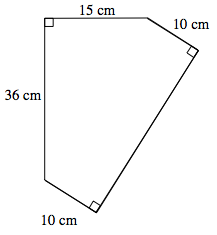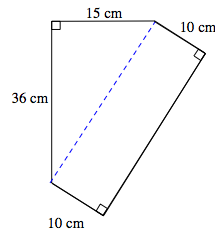### Home > MC2 > Chapter 9 > Lesson 9.3.4 > Problem9-123

9-123.
1. The figure shown below is made up of a right triangle and a rectangle. Homework Help ✎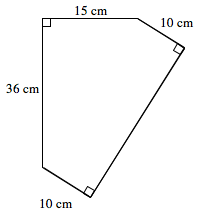1. Copy the shape on your paper. Then draw a line to help divide the figure into two separate shapes.

2. Use the dimensions given to find the area of the triangle, the rectangle, and the whole figure. Be sure to show all of your subproblems.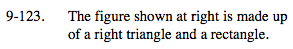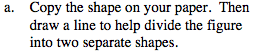Where can you draw a dividing line so that a rectangle
and a triangle are formed?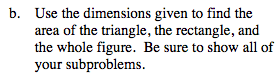We need to know the length of the dividing line in order to find the area of the rectangle.
The line is also the hypotenuse for the triangle.
How can we find the length of the hypotenuse of a triangle if we are given both legs?